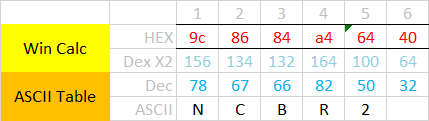# ASCII number to Byte.

• Hello, this is my first post on forum.
Here is what i have to do:
i have a QString. example: "EARTH"
the i need take every char, one by one, convert to ascii number, multiply the number by 2, convert back again to ascii, and build a byte array to send over tcpsocket.

rules:
this bytearray is for a ax25 header, his lenght is always 6. if i receive some string smaller then 6, i have to fill the rest with "".

my first try was:
build a byteArray_out filled with 0x40 (thats represents "" ).

1. do a loop (at the satId.at.size() representing the "in" data) and take the chars from satId, and convert to ascii number, finally multiplying by 2 (ok).
int v = (satId.at(i).toLatin1())*2;

2. build a bytearray from this number, take the first byte of this bytearray, and write in byteArray_out position at the loop (didnt work)

byteArray_out [i] = QByteArray::number(v, 16);

I build a code taking another way (but i belive is not the better way){

``````int AX25Header::Initialize(QString satId, QString callsign)
{
if(satId.size() > 6 || callsign.size() > 6)
return -1;
//converting formula = string to ASCII decimal, decimal X2, new decimal to hex.
//0x40 = ""

QString satIdx2;
for(int i=0; i < 6; i++){
if(i < satId.size()){
int v = satId.at(i).toLatin1();
v = v*2;
}
else
satIdx2.append("40");
}
QByteArray satId_ba = QByteArray::fromHex(satIdx2.toUtf8());
if(satId_ba.size()!=6){
log("destination callsign size error");
return -1;
}
m_Instance.setDestinationCallSign(satId_ba);
m_Instance.setDestinationSSID(0x00);
QString satId_ba_str = satId_ba.toHex();
log("TC AX25 Destination Callsign = " + satId_ba_str);

QString callsignx2;
for(int i=0; i < 6; i++){
if(i < callsign.size()){
int v = callsign.at(i).toLatin1();
v = v*2;
}
else
callsignx2.append("40");
}
QByteArray callsignx2_ba = QByteArray::fromHex(callsignx2.toUtf8());
if(callsignx2_ba.size()!=6){
log("source callsign size error");
return -1;
}
m_Instance.setSourceCallSign(callsignx2_ba);
m_Instance.setSourceSSID(0x00);
QString callsign_ba_str = callsignx2_ba.toHex();
log("TC AX25 Source Callsign = " + callsign_ba_str);

// AX25 Control Fields
m_Instance.setAX25ControlField(0x03);
m_Instance.setAX25PIDField(0xF0);

return 0;
}
``````

• Hi and welcome to devnet,

First thing to do: use only QByteArray. QString stores your string in UTF16 and you are doing useless back and forth transformation.

What do you mean by ASCII number ? Every char has a numerical representation so I am not sure what you want to achieve here.

• @SGaist said in ASCII number to Byte.:

Every char has a numerical representation so I am not sure what you want to achieve here.

ill give you a didatic example.

i have this string:
N C B R 2

i have to convert every char to a ascii number

N = 78
C = 76
B = 66
R = 82
2 = 50
"" = 32

then i have to multiply the ascii numbers by 2:

78 -> 156
132 -> 134
66 -> 132
82 -> 50
50 -> 100
32 -> 64

and finally convert the new numbers to hex:

156 -> 9c
132 -> 86
132 -> 84
164 -> a4
100 -> 64
64 -> 40

so my final QByteArray is "9c-86-84-a4-64-40"

Look this table, the conversion is down to up.• When you've only ascii characters in your string then convert your QString to a QByteArray and iterate over the single chars. Converting an integer back to a QString / QByteArray can be done with QString/QByteArray::number()